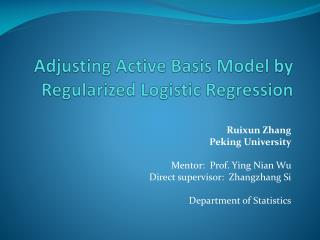Download PresentationAdjusting Active Basis Model by Regularized Logistic Regression

# Adjusting Active Basis Model by Regularized Logistic Regression

Download Presentation## Adjusting Active Basis Model by Regularized Logistic Regression

- - - - - - - - - - - - - - - - - - - - - - - - - - - E N D - - - - - - - - - - - - - - - - - - - - - - - - - - -
##### Presentation Transcript

1. Adjusting Active Basis Model by Regularized Logistic Regression Ruixun Zhang Peking University Mentor: Prof. Ying Nian Wu Direct supervisor: Zhangzhang Si Department of Statistics

2. Outline • Active Basis model as a generative model • Supervised and unsupervised learning • Hidden variables and maximum likelihood • Discriminative adjustment after generative learning • Logistic regression, SVM and AdaBoost • Over-fitting and regularization • Experiment results

3. Active Basis – Representation • An active basis consists of a small number of Gabor wavelet elements at selected locations and orientations

4. Active Basis – Learning and Inference • Shared sketch algorithm • Local normalization • measures the importance of Bi • Inference: matching the template at each pixel, and select the highest score.

5. Active Basis – Example

6. General Problem – Unsupervised Learning • Unknown categories – mixture model • Unknown locations and scales • Basis perturbations ……………… • Active plates – a hierarchical active basis model Hidden variables

7. Starting from Supervised Learning • Data set: head_shoulder, 131 positives, 631 negatives. ………………

8. Active Basis as a Generative Model • Active basis – Generative model • Likelihood-based learning and inference • Discover hidden variables – important for unsupervised learning. • NOT focus on classification task (no info from negative examples.) • Discriminative model • Not sharp enough to infer hidden variables • Only focus on classification • Over-fitting.

9. Discriminative Adjustment • Adjust λ’s of the template • Logistic regression – consequence of generative model • Loss function: p

10. Logistic Regression Vs. Other Methods Logistic regression SVM AdaBoost Loss

11. Problem: Over-fitting • head_shoulder; svm from svm-light, logistic regression from matlab. • template size 80, training negatives 160, testing negatives 471. • active basis • active basis + logistic regression • active basis + SVM • active basis + AdaBoost

12. Regularization for Logsitic Regression • Loss function for • L1-regularization • L2-regularization • Corresponding to a Gaussian prior • Regularization without the intercept term

13. Experiment Results • head_shoulder; svm from svm-light, L2-logistic regression from liblinear. • template size 80, training negatives 160, testing negatives 471. • active basis • active basis + logistic regression • active basis + SVM • active basis + AdaBoost • Tuning parameter C=0.01.

14. With or Without Local Normalization • All settings same as the head_shoulder experiment WithWithout

15. Tuning Parameter All settings the same. Change C, see effect of L2-regularization

16. Experiment Results – More Data • horses; svm from svm-light, L2-logistic regression from liblinear. • template size 80, training negatives 160, testing negatives 471. • active basis • active basis + logistic regression • active basis + SVM • active basis + AdaBoost • Dimension reduction by active basis, so speed is fast. • Tuning parameter C=0.01.

17. Experiment Results – More Data • guitar; svm from svm-light, L2-logistic regression from liblinear. • template size 80, training negatives 160, testing negatives 855. • active basis • active basis + logistic regression • active basis + SVM • active basis + AdaBoost • Dimension reduction by active basis, so speed is fast. • Tuning parameter C=0.01.

18. Future Work • Extend to unsupervised learning – adjust mixture model • Generative learning by active basis • Hidden variables • Discriminative adjustment on feature weights • Tighten up the parameters, • Improve classification performances • Adjust active plate model

19. Acknowledgements • Prof. Ying Nian Wu • Zhangzhang Si • Dr. Chih-Jen Lin • CSST program

20. Refrences • Wu, Y. N., Si, Z., Gong, H. and Zhu, S.-C. (2009). Learning Active Basis Model for Object Detection and Recognition. International Journal of Computer Vision. • R.-E. Fan, K.-W. Chang, C.-J. Hsieh, X.-R. Wang, and C.-J. Lin. (2008). LIBLINEAR: A Library for Large Linear Classification. Journal of Machine Learning Research. • Lin, C. J., Weng, R.C., Keerthi, S.S. (2008). Trust Region Newton Method for Large-Scale Logistic Regression. Journal of Machine Learning Research. • Vapnik, V. N. (1995). The Nature of Statistical Learning Theory. Springer. • Joachims, T. (1999). Making large-Scale SVM Learning Practical. Advances in Kernel Methods - Support Vector Learning, B. Schölkopf and C. Burges and A. Smola (ed.), MIT-Press. • Freund, Y. and Schapire, R. E. (1997). A Decision-Theoretic Generalization of On-Line Learning and an Application to Boosting. Journal of Computer and System Sciences. • Viola, P. and Jones, M. J. (2004). Robust real-time face detection. International Journal of Computer Vision. • Rosset, S., Zhu, J., Hastie, T. (2004). Boosting as a Regularized Path to a Maximum Margin Classifier. Journal of Machine Learning Research. • Zhu, J. and Hastie, T. (2005). Kernel Logistic Regression and the Import Vector Machine. Journal of Computational and Graphical Statistics. • Hastie, T., Tibshirani, R. and Friedman, J. (2001) Elements of Statistical Learning; Data Mining, Inference, and Prediction. New York: Springer. • Bishop, C. (2006). Pattern Recognition and Machine Learning. New York: Springer. • L. Fei-Fei, R. Fergus and P. Perona. (2004). Learning generative visual models from few training examples: an incremental Bayesian approach tested on 101 object categories. IEEE. CVPR, Workshop on Generative-Model Based Vision. • Friedman, J., Hastie, T. and Tibshirani, R. (2000). Additive logistic regression: A statistical view of boosting (with discussion). Ann. Statist.

21. Thank you.Q & A